The DCT transforms a signal from a spatial representation into a frequency representation. In an image, most of the energy will be concentrated in the lower frequencies, so if we transform an image into its frequency components and throw away the higher frequency coefficients, we can reduce the amount of data needed to describe the image without sacrificing too much image quality.

A simplified JPEG compressor:

• Cut an image up into blocks of 8x8 pixels
• Run each block through an 8x8 2D DCT
• Quantize the resulting coefficients (i.e. throw away unimportant information to reduce the filesize - JPEG does this by dividing the coefficients by a quantization matrix in order to get long runs of zeros)
• Compress the quantized coefficients using a lossless method (RLE, Huffman, Arithmetic coding, etc)

Wikipedia has an excellent article about the discrete cosine transform. The rest of this page describes a two-dimensional DCT-II and inverse DCT and gives implementations in C.

Given an image, $$S$$, in the spatial domain, the pixel at coordinates $$(x,y)$$ is denoted $$S_{yx}$$. To transform $$S$$ into an image in the frequency domain, $$F$$, we can use the following:

$$\begin{eqnarray*} C_u & = & \left\{\begin{array}{ll} \frac{1}{\sqrt{2}} & \textrm{if u=0}\\ 1 & \textrm{else} \end{array}\right.\\ C_v & = & \textrm{(similar to the above)}\\ F_{vu} & = & \frac{1}{4} C_v C_u \sum_{y=0}^{N-1} \sum_{x=0}^{N-1} S_{yx} cos\left(v\pi\frac{2y+1}{2N}\right) cos\left(u\pi\frac{2x+1}{2N}\right) \end{eqnarray*}$$

Four example blocks in spatial and frequency domains: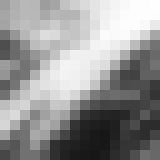> > >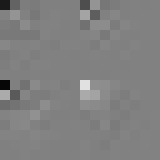Spatial Frequency

# Inverse Discrete Cosine Transform

To rebuild an image in the spatial domain from the frequencies obtained above, we use the IDCT:

$$S_{yx} = \frac{1}{4} \sum_{v=0}^{N-1} \sum_{u=0}^{N-1} C_v C_u F_{vu} cos\left(v\pi\frac{2y+1}{2N}\right) cos\left(u\pi\frac{2x+1}{2N}\right)$$

Mathematically, the DCT is perfectly reversable and we do not lose any image definition until we start quantizing coefficients. In my simulation, I simply threw most of them out. A better quantizer would decrease precision gradually instead of simply zeroing out components.

Below is the original image and reconstructions of it using only the most significant $$n \times n$$ coefficients.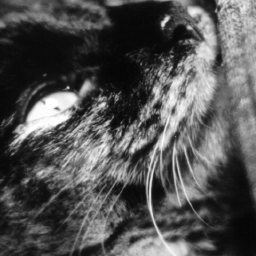Original image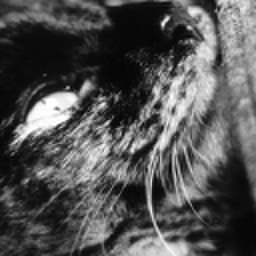4x4 (25%)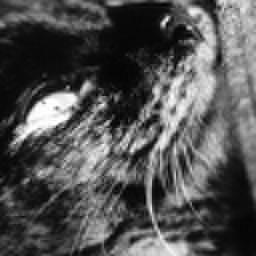3x3 (14%)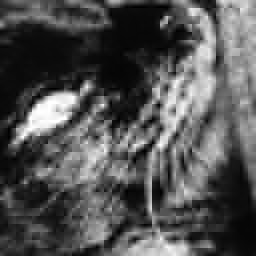2x2 (6%)

Do those artifacts look familiar?

You can find slow DCT/IDCT code in listing1.c. Note that you might also want the Targa reader/writer code.

# Optimization

The implementation above uses four nested loops and has complexity $$\mathrm{O}\left(n^4\right)$$ for a 2D DCT of size $$n \times n$$. We can do better by using row-column decomposition: build a 2D DCT by running a 1D DCT over every row and then every column.

The 1D DCT-II is:

$$F_{u} = \frac{1}{2} C_u \sum_{x=0}^{N-1} S_{x} cos\left(u\pi\frac{2x+1}{2N}\right)$$

listing2.c uses a 1D DCT with complexity $$\mathrm{O}\left(n^2\right)$$, running it $$2n$$ times to build a 2D DCT with complexity $$\mathrm{O}\left(n^3\right)$$.

We can do better again by replacing the naive $$\mathrm{O}\left(n^2\right)$$ DCT algorithm with one factored similarly to a Fast Fourier Transform which would have $$\mathrm{O}\left(n \log n\right)$$ complexity.

The AAN (Arai/Agui/Nakajima) algorithm is one of the fastest known 1D DCTs. listing3.c shows a faster 2D DCT built from 1D AAN DCTs.